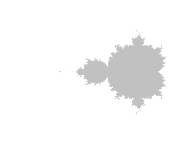# mandelbrot

Curious whether your favourite complex number is a member of the Mandelbrot set?

—No? Then probably you just want to draw pretty pictures.

Install with:

``devtools::install_github("blmoore/mandelbrot")``

## Examples

``````library(mandelbrot)

mb <- mandelbrot()
plot(mb)``````

Pretty colours:

``````mb <- mandelbrot(xlim = c(-0.8438146, -0.8226294),
ylim = c(0.1963144, 0.2174996),
iterations = 500, resolution = 800)

cols <- mandelbrot_palette(RColorBrewer::brewer.pal(11, "Spectral"), fold = FALSE)
plot(mb, col = cols)``````

Experiment with transforms:

``````# layout config (reset after)
par(mfrow = c(1, 2), pty = "s", mar = rep(0, 4))
plot(mb, col = cols, transform = "inverse")
plot(mb, col = cols, transform = "log")``````

Don’t like `image`? Convert to a `data.frame` and use with ggplot2:

``````library(ggplot2)

mb <- mandelbrot(xlim = c(-0.8335, -0.8325),
ylim = c(0.205, 0.206),
resolution = 1200L,
iterations = 1000)

# weird uneven palette made for a heatmap viz:
# https://benjaminlmoore.wordpress.com/2015/04/09/recreating-the-vaccination-heatmaps-in-r/
cols <- c(
colorRampPalette(c("#e7f0fa", "#c9e2f6", "#95cbee",
"#0099dc", "#4ab04a", "#ffd73e"))(10),
colorRampPalette(c("#eec73a", "#e29421", "#e29421",
"#f05336","#ce472e"), bias=2)(90),
"black")

df <- as.data.frame(mb)
ggplot(df, aes(x = x, y = y, fill = value)) +
geom_raster(interpolate = TRUE) + theme_void() +
scale_fill_gradientn(colours = cols, guide = "none") ``````

`mandelbrot0` is a faster interface for `as.data.frame(mandelbrot(...))`.